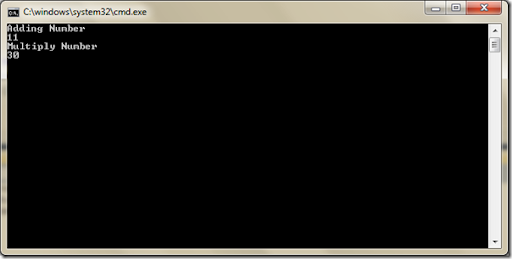Friday, April 13, 2012

## Multicast delegates in c#

In yesterday’s post We learn about Delegates and how we can use delegates in C#. In today’s blog post we are going to learn about Multicast delegates.

### What is Multicast Delegates?

As we all know we can assign methods as object to delegate and later on we can call that method with the help delegates. We can also assign more then methods to delegates that is called Multicast delegates. It’s provide functionality to execute more then method at a time. It’s maintain delegates as invocation list (linked list).

Let’s understands that via a example. We are going to use yesterday’s example and then we will extend that code multicast delegates. Following code I have written to demonstrate the multicast delegates.

```using System;
namespace Delegates
{
class Program
{
public delegate void CalculateNumber(int a, int b);
static void Main(string[] args)
{
int a = 5;
int b = 5;
CalculateNumber multiplyNumber = new CalculateNumber(MultiplyNumber);
CalculateNumber multiCast = (CalculateNumber)Delegate.Combine (addNumber, multiplyNumber);
multiCast.Invoke(a,b);
}
public static void AddNumber(int a, int b)
{
Console.WriteLine(5 + 6);
}
public static void  MultiplyNumber(int a, int b)
{
Console.WriteLine("Multiply Number");
Console.WriteLine(5 * 6);
}
}
}
```
As you can see in the above code I have created two method one for adding two numbers and another for multiply two number. After that I have created two same CalculateNumber delegates addNumber and multiplyNumber then I have create a multicast delegates multiCast with combining two delegates. Now I want to call this both method so I have used Invoke method to call this delegates.

As now our code is let’s run the application. Following is a output as expected.As you can we can execute multiple methods with multicast delegates the only thing you need to take care is that we need to type for both delegates. That’s it. Hope you like it. Stay tuned for more.. Till then happy
programming.Share: